Next: Examples of Wavelets Up: The Wavelet Transform Previous: Introduction

The continuous wavelet transform

The Morlet-Grossmann definition of the continuous wavelet transform  for a 1D signal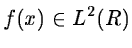is:(14.1)

where z* denotes the complex conjugate of z,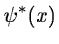is the analyzing wavelet, a (>0) is the scale parameter and b is the position parameter. The transform is characterized by the following three properties:
1.
it is a linear transformation,
2.
it is covariant under translations:(14.2)

3.
it is covariant under dilations:(14.3)

The last property makes the wavelet transform very suitable for analyzing hierarchical structures. It is like a mathematical microscope with properties that do not depend on the magnification.

In Fourier space, we have: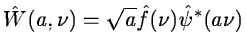(14.4)

When the scale a varies, the filter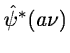is only reduced or dilated while keeping the same pattern.

Now consider a function W(a,b) which is the wavelet transform of a given function f(x). It has been shown [#grossmann<#14252,#holschn<#14253] that f(x) can be restored using the formula: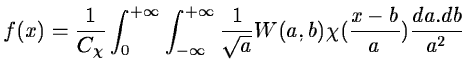(14.5)

where:(14.6)

Generally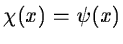, but other choices can enhance certain features for some applications.

The reconstruction is only available if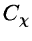is defined (admissibility condition). In the case of, this condition implies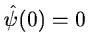, i.e. the mean of the wavelet function is 0.Next: Examples of Wavelets Up: The Wavelet Transform Previous: Introduction
Petra Nass
1999-06-15